# SSC EXAMS | Quantitative Aptitude Practice Questions (Day-26)

Dear Aspirants, Here we have given the Important SSC Exam 2019 Practice Test Papers. Candidates those who are preparing for SSC 2019 can practice these questions to get more confidence to Crack SSC 2019 Examination.

[WpProQuiz 5254]

1) A farmer owns a circular plot with diameter 56m. He builds a 7m wide garden around the plot outside which costs him Rs.260 per square meter. What is the total cost borne by the farmer for building the entire garden?

a) Rs.416550

b) Rs.408220

c) Rs.360360

d) Rs.228700

2) A man invited some boys and girls on his birthday. The number of boys was less than that of girls by 2. He gave Rs.10 to each boy and Res. 20 to each girl. If the total amount distributed by him is Rs.280, what was the number of boys?

a) 25

b) 15

c) 10

d) 8

3) A sales executive’s commission is 5% on all sale up to Rs.10000 and 4% on all sales exceeding this. He remits Rs.31100 to his parent company after deducting his commission. His sales was worth

a) Rs.36100

b) Rs.35100

c) Rs.35000

d) Rs.32500

4)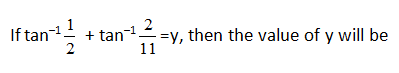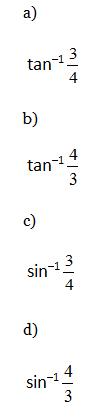5) If 45% of the total students of class have offered Mathematics and 85% offered Biology. Find the percentage of students of class who have offered Biology only not Mathematics.

a) 30%

b) 55%

c) 60%

d) 45%

6) 25 L of wine has 20% alcohol and 80% distilled water. Mayank and his friends take out 5 L of wine and add 5 L of pure alcohol to it. Next day they again take out 5 L of wine and add 5 L of pure alcohol. Third day they repeat to process but this time add 5 L of pure distilled water. What is the final volume of alcohol in the wine?

a) 10.87 L

b) 9.76 L

c) 10.11 L

d) 12.8 L

7) The average marks of students in three sections A, B and C are 10, 20 and 30 respectively. If the average marks of all the students in section B and C together is 25 and the average marks of all the students in sections A and B together is 16 2/3. Find the average marks of all the three classes put together.

a) 22.11

b) 22

c) 22.5

d) 23

8) Purnendu’s salary is first increased by 25% and then decreased by 20%. The result is the same as Sanjay’s salary increased by 20% and then reduced by 25%, find the ratio of Sanjay’s salary to that of Purnendu?

a) 4 : 3

b) 11 : 10

c) 10 : 9

d) 12 : 11

9) Four fifth of the votes in Patna promised to vote for Satrughan Sinha and the rest promised to vote for Shekhar. Of these voters, 10% of the voters who had promised to vote for Satrughan Sinha didn’t vote on Election Day. While 20% of the voters who had promised to vote for Shekhar didn’t vote on the Election Day. What is the total no. of votes polled, if Satrughan Sinha got 216 votes?

a) 200

b) 300

c) 264

d) 600

10) A plastic cube dropped in water is found to float with its base perfectly horizontal. If exactly one-third of the total surface of cube is submerged in water then what fraction of volume of the cube is above the surface of water?

a) 0.66

b) 0.875

c) 0.525

d) 0.75

Radius of the circular plot = 56/2 = 28 m

Radius of the plot with the garden = 28 m + 7m = 35m

Area of the circular plot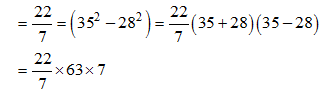Total cost borne by the farmer for building the entire garden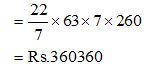Let the number of boys invited on birthday = x

The number of girls invited on birthday = x + 2

According to question,

10x + 20(x + 2) = 280

10x = 20x + 40 = 280

30x = 280 – 40

30x = 240

x = 240/ 30

x = 8

Let sales be Rs. (10000 + x)

Then, Executive’s Commission = 500 + 0.04x

According to question,

10000 + x – 500 – 0.04x = 31100

9500 + 0.96x = 31100

0.96x = 21600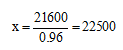Total sales = 10000 + 22500 = Rs.32500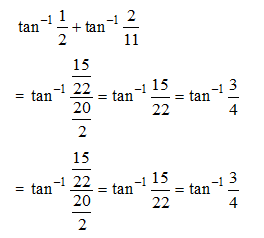n (M B) = n (M) + n (B) – n (M B)

100 = 45 + 85 – n (M  B)

n (M  B) = 130 – 100 = 30%

Only n (B) = n (B) – n (M  B) = 85 – 30 = 55%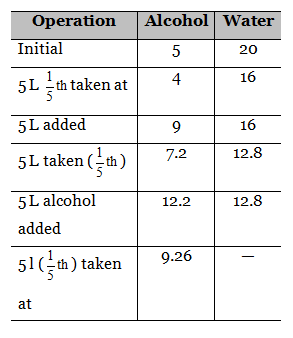Hence, 9.76 is the quantity alcohol remains.

Average of A = a = 10

b = 20

c = 30

Let na, nb and nc be the number of students is each classes given that

Average of B and C = 25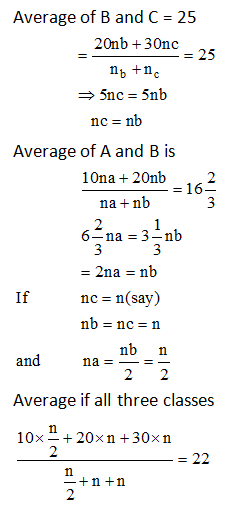Let Purnendu’s salary be Rs. 100.

After 25% increments, it will become Rs. 125% again, after 20% decrement, it will become Rs. 100. Now, taking Sanjay’s salary to be Rs. 100

After 20% increments, it will become Rs. 120

After 25% decrement, new salary will become Rs. 90. So 100 R = 90 K

K/R = 100/90

Ratio if Sanjay’s to Purnendu’s salary is 10 : 9

Let the total number of voters be ‘X’ then 4/5th of the votes.

Polled will be given to Satrughan Sinha, and rest i.e., 1/5th is been polled to Shekhar Again

10% of 4x/5th of the voters polled Satrughan Sinha

Now, Satrughan Sinha got 216 votes

18x/25 = 216

X = 25 × 216 / 18 = 300

Shekar got 1x/5 of the total votes out of these voters only

80% voted, so shekar will get

80/ 100 × (x/5) = 7x/25

Satrughan Sinha got 216 votes i.e. 18x/25 = 216

X = 300

300 voters were these allover out of which 10% for satrughan Sinha and20% for Shekar did not vote,

Votes polled = 300 = 3×300/25 =264

Let the area of each face = A sq unit

So, total surface area = 6A

Hence, submerged area = 1/3 (6A) = 2A

So four vertical sides have A sq. unit under water, ie each vertical face has ¼ of its height under water so volume of cube under water = ¼ of volume

And volume of cube above the water = ¾ of volume

Hence, required fraction = ¾ = 0.75

### *******************

#### SSC CGL EXAMS 2019 |Quantitative Aptitude Practice Questions (Day-23)